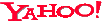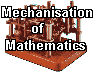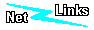General Information
 GAMS : Guide to Available Mathematical Software - a cross-index and virtual repository of mathematical and statistical software components of use in computational science and engineering. LMS Journal of Computation and Mathematics SymbolicNet a general resource on Symbolic and Algebraic computer systens, including a SAC Systems Listing
Mathematical Software
 MathWorks - vendor of Matlab & Simulink, a comprehensive environment for numerical mathematics and a simulation tool using models presented as diagrams. See also: mathools.net Waterloo Symbolic Computation Group undertakes research and development of algorithms for computer algebra, including both symbolic computation and hybrid symbolic-numeric computation. The algorithms developed are incorporated into the MAPLE computer algebra system. MACSYMA "the world's first, and still best, artificial intelligence based math engine providing easy to use, powerful math software for symbolic and numeric computing"
 Mathematica - Stephen Wolfram's world beating symbolic mathematics software. See also: The Mathematica Website, The Mathematica Journal, MathSource Wolfram Research. MATRIXx - graphical modelling and simulation from ISI Magma- designed to solve computationally hard problems in algebra, number theory, geometry and combinatorics. MuPAD - "the only truly open computer algebra system." Reduce
OpenMath
a standard for communicating mathematical objects between computer programs
HomePage
Introduction
North American OpenMath Initiative
SGML
standard generalised markup language
W3C XML activity
XML
Math HTML
Math HTML overview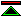©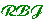created 1995-10-21 modified 2001-10-18